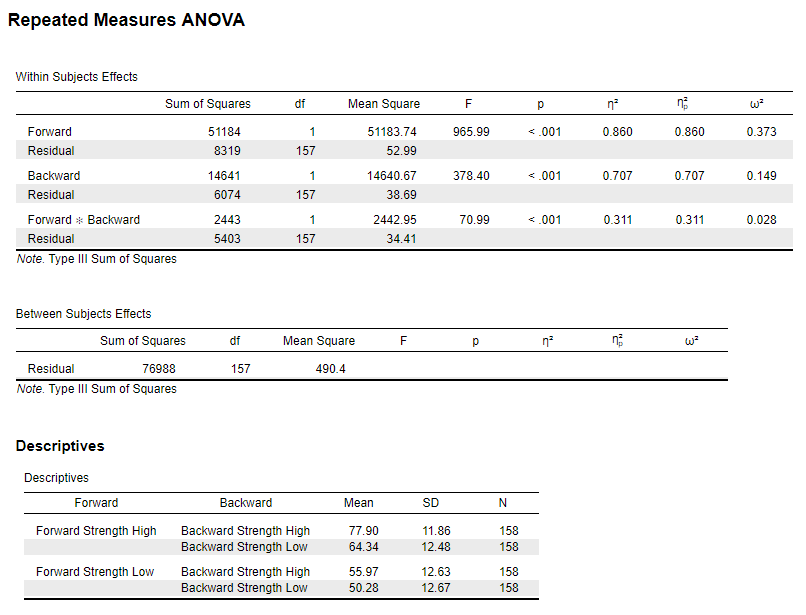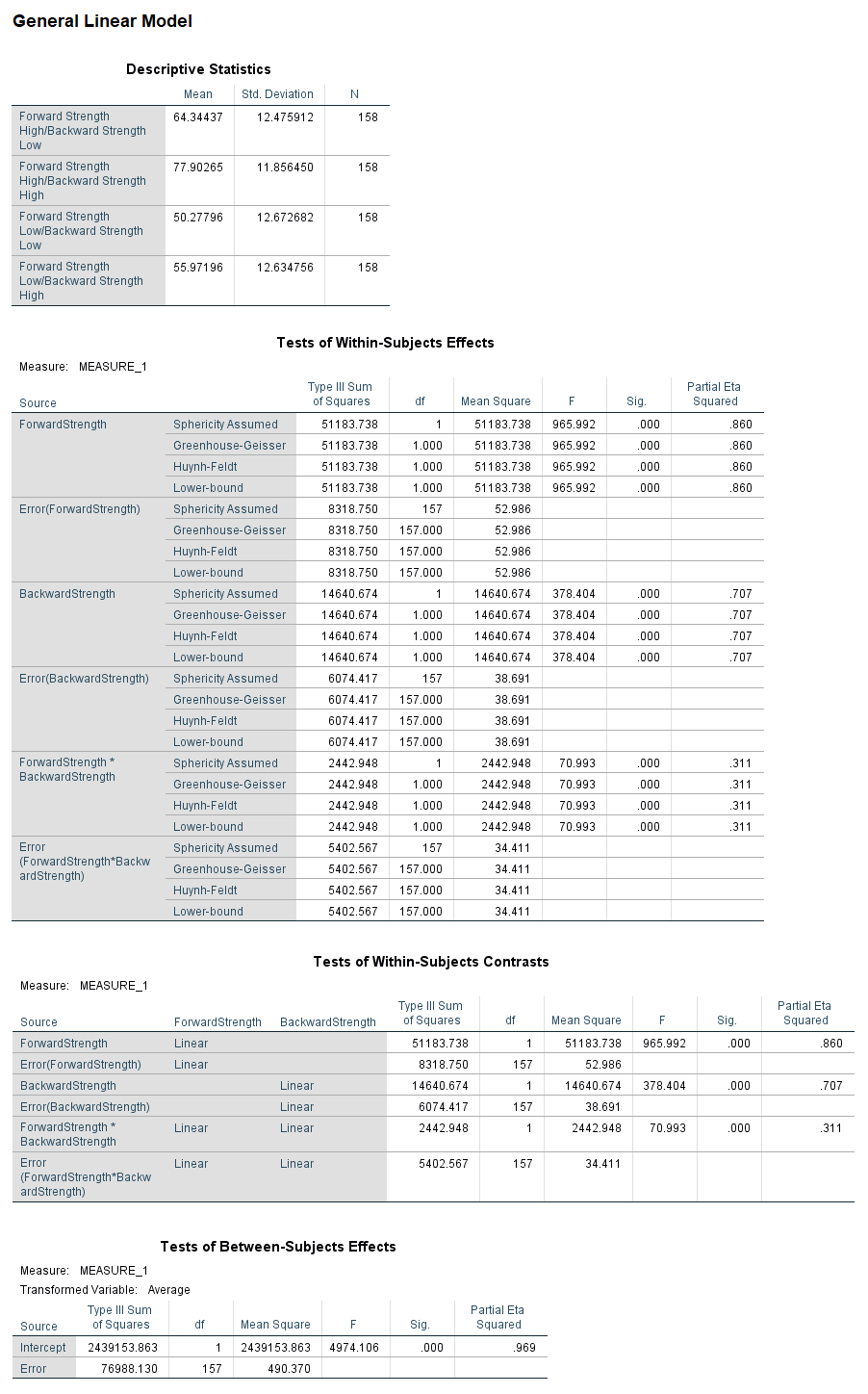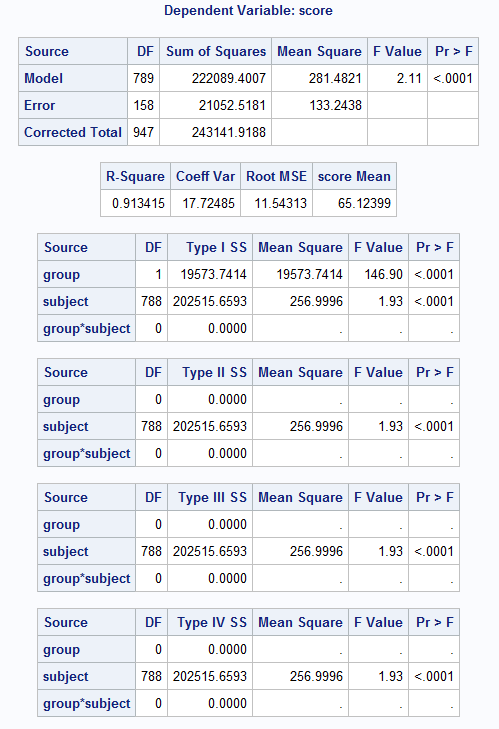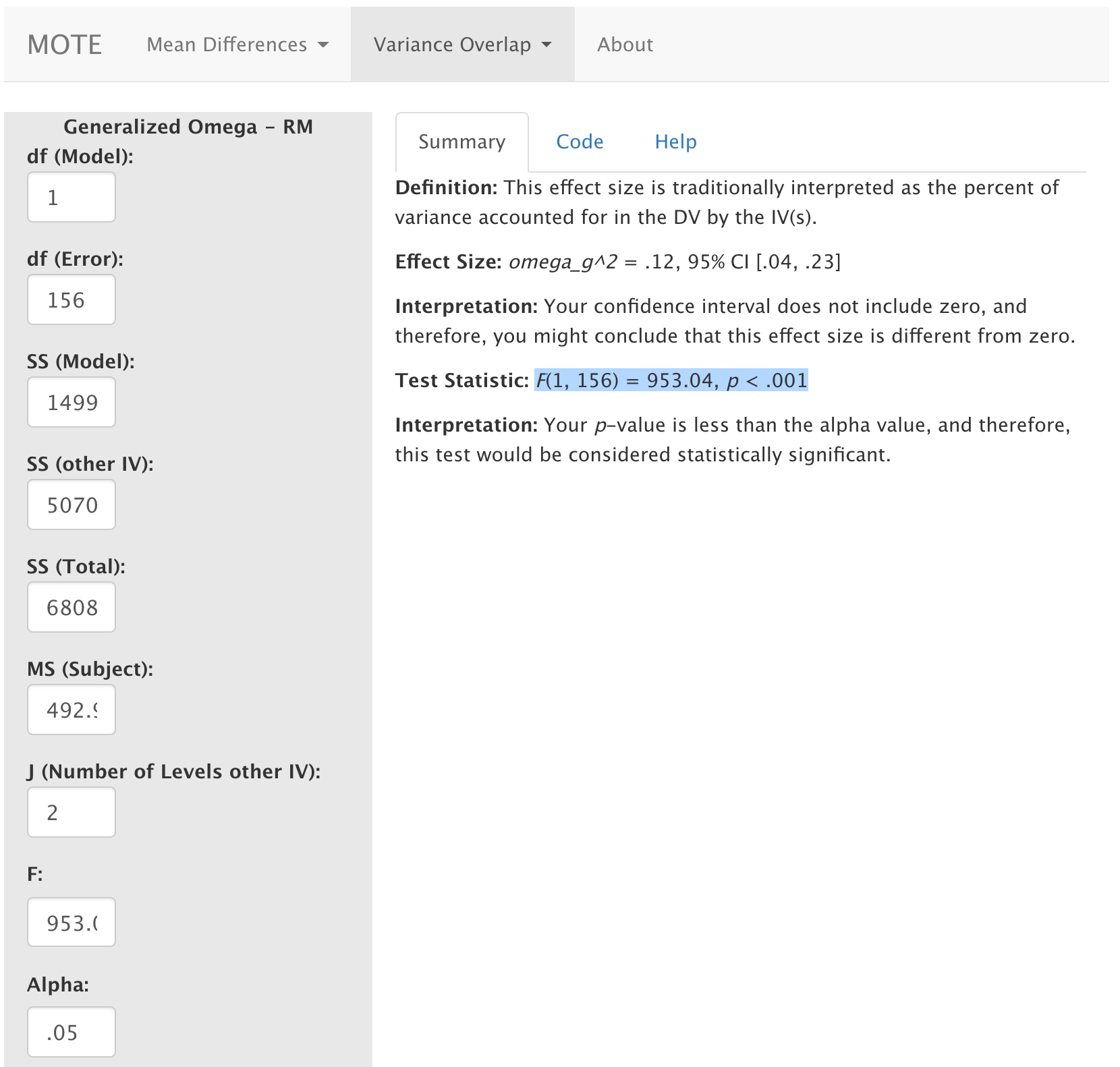# Description

This function displays generalized omega squared from ANOVA analyses and its non-central confidence interval based on the F distribution. These values are calculated directly from F statistics and can be used for between subjects and repeated measures designs. Remember if you have two or more IVs, these values are partial omega squared.

The formula for $\omega_G^2$ is: $$\frac{SS_{modelA} - (df_{modelA} \times MS_{subjectA})} {SS_{total} + SS_{modelB} + J_B \times MS_{subjectA}}$$

Note: A is the target IV to calculate generalized omega squared on. B is the other IV. J is the number of levels for IV B.

# R Function

omega.gen.SS.rm(dfm, dfe, ssm, ssm2, sst, mss, j, Fvalue, a = 0.05)

# Arguments

• dfm = degrees of freedom for the model/IV/between
• dfe = degrees of freedom for the error/residual/within
• ssm = sum of squares for the MAIN model/IV/between
• ssm2 = sum of squares for the OTHER model/IV/between
• sst = sum of squares total across the whole ANOVA
• mss = mean square for the subject variance
• j = number of levels in the OTHER IV
• Fvalue = F statistic from the output for your IV
• a = significance level

# Example

In this experiment people were given word pairs to rate based on their “relatedness”. How many people out of a 100 would put LOST-FOUND together? Participants were given pairs of words and asked to rate them on how often they thought 100 people would give the second word if shown the first word. The strength of the word pairs was manipulated through the actual rating (forward strength: FSG) and the strength of the reverse rating (backward strength: BSG). Is there an interaction between FSG and BSG when participants are estimating the relation between word pairs? The data are available on GitHub. Example output from JASP, SPSS, and SAS are shown below.

JASPSPSSSAS# Function in R:

omega.gen.SS.rm(dfm = 1, dfe = 156, ssm = 14994.636, ssm2 = 50700.145, sst = 68084.034, mss = 492.999, j = 2, Fvalue = 953.042, a = 0.05)

# MOTE

## Screenshot## Effect Size:

$\omega_g^2$ = .12, 95% CI [.04, .23]

## Interpretation:

Your confidence interval does not include zero, and therefore, you might conclude that this effect size is different from zero.

Not applicable.

## Test Statistic:

F(1, 156) = 953.04, p < .001

## Interpretation:

Your p-value is less than the alpha value, and therefore, this test would be considered statistically significant.